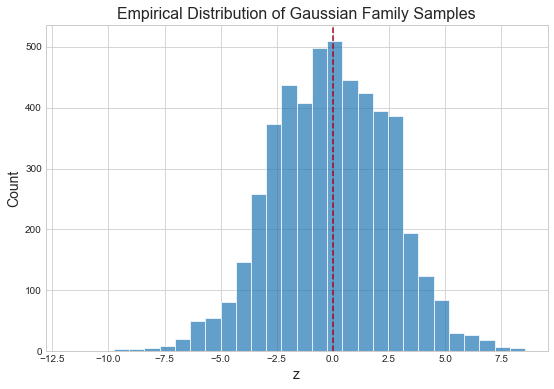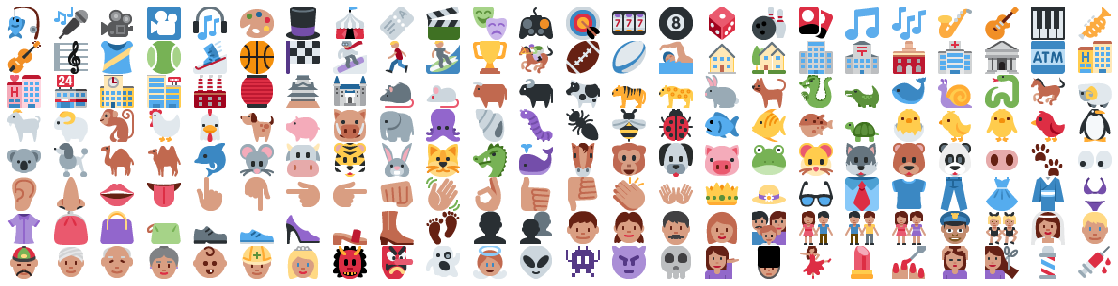# Neurally Embedded Emojis¶

As I move through my 20's I'm consistently delighted by the subtle ways in which I've changed.

• Will at 22: Reggaeton is a miserable, criminal assault to my ears.
• Will at 28: Despacito (Remix) for breakfast, lunch, dinner.
• Will at 22: Western Europe is boring. No — I've seen a lot of it! Everything is too clean, too nice, too perfect for my taste.
• Will at 28, in Barcelona, after 9 months in Casablanca: Wait a second: I get it now. What is this summertime paradise of crosswalks, vehicle civility and apple-green parks and where has it been all my life?
• Will at 22: Emojis are weird.
• Will at 28: 🚀 🤘 💃🏿 🚴🏻 🙃.

Emojis are an increasingly-pervasive sub-lingua-franca of the internet. They capture meaning in a rich, concise manner — alternative to the 13 seconds of mobile thumb-fumbling required to capture the same meaning with text. Furthermore, they bring two levels of semantic information: their context within raw text and the pixels of the emoji itself.

The original aim of this post was to explore Siamese question-answer models of the type typically applied to the InsuranceQA Corpus as introduced in "Applying Deep Learning To Answer Selection: A Study And An Open Task" (Feng, Xiang, Glass, Wang, & Zhou, 2015). We'll call them SQAM for clarity. The basic architecture looks as follows:By layer and in general terms:

1. An input — typically a sequence of token ids — for both question (Q) and answer (A).
2. An embedding layer.
3. Convolutional layer(s), or any layers that extract features from the matrix of embeddings. (A matrix, because the respective inputs are sequences of token ids; each id is embedded into its own vector.)
4. A max-pooling layer.
5. A tanh non-linearity.
6. The cosine of the angle between the resulting, respective embeddings.

### As canonical recommendation¶

Question answering can be viewed as canonical recommendation: embed entities into Euclidean space in a meaningful way, then compute dot products between these entities and sort the list. In this vein, the above network is (thus far) quite similar to classic matrix factorization yet with the following subtle tweaks:

1. Instead of factorizing our matrix via SVD or OLS we build a neural network that accepts (question, answer), i.e. (user, item), pairs and outputs their similarity. The second-to-last layer gives the respective embeddings. We train this network in a supervised fashion, optimizing its parameters via stochastic gradient descent.
2. Instead of jumping directly from input-index (or sequence thereof) to embedding, we first compute convolutional features.

In contrast, the network above boasts one key difference: both question and answer, i.e. user and item, are transformed via a single set of parameters — an initial embedding layer, then convolutional layers — en route to their final embedding.

Furthermore, and not unique to SQAMs, our network inputs can be any two sequences of (tokenized, max-padded, etc.) text: we are not restricted to only those observed in the training set.

## Question-emoji models¶

Given my accelerating proclivity for the internet's new alphabet, I decided to build text-question-emoji-answer models instead. In fact, this setup gives an additional avenue for prediction: if we make a model of the answers (emojis) themselves, we can now predict on, i.e. compute similarity with, each of

1. Emojis we saw in the training set.
2. New emojis, i.e. either not in the training set or new (like, released months from now) altogether.
3. Novel emojis generated from the model of our data. In this way, we could conceivably answer a question with: "we suggest this new emoji we've algorithmically created ourselves that no one's ever seen before."

Let's get started.

## Convolutional variational autoencoders¶

Variational autoencoders are comprised of two models: an encoder and a decoder. The encoder embeds our 872 emojis of size $(36, 36, 4)$ into a low-dimensional latent code, $z_e \in \mathbb{R}^{16}$, where $z_e$ is a sample from an emoji-specific Gaussian. The decoder takes as input $z_e$ and produces a reconstruction of the original emoji. As each individual $z_e$ is normally distributed, $z$ should be distributed normally as well. We can verify this with a quick simulation.

In :
import matplotlib.pyplot as plt
import numpy as np

%matplotlib inline
plt.style.use('seaborn-whitegrid')

In :
mu = np.linspace(-3, 3, 10)
sd = np.linspace(0, 3, 10)
z_samples = []

for m in mu:
for s in sd:
samples = np.random.normal(loc=m, scale=s, size=50)
z_samples.append( samples )

z_samples = np.array(z_samples).ravel()

plt.figure(figsize=(9, 6))
plt.hist(z_samples, edgecolor='white', linewidth=1, bins=30, alpha=.7)
plt.axvline(0, color='#A60628', linestyle='--')
plt.xlabel('z', fontsize=14)
plt.ylabel('Count', fontsize=14)
plt.title('Empirical Distribution of Gaussian Family Samples', fontsize=16)

Out:
<matplotlib.text.Text at 0x113c318d0>Training a variational autoencoder to learn low-dimensional emoji embeddings serves two principal ends:

1. We can feed these low-dimensional embeddings as input to our SQAM.
2. We can generate novel emojis with which to answer questions.

As the embeddings in #1 are multivariate Gaussian, we can perform #2 by passing Gaussian samples into our decoder. We can do this by sampling evenly-spaced percentiles from the inverse CDF of the aggregate embedding distribution:

percentiles = np.linspace(0, 1, 20)
for p in percentiles:
z = norm.ppf(p, size=16)
generated_emoji = decoder.predict([z])


NB: norm.ppf does not accept a size parameter; I believe sampling from the inverse CDF of a multivariate Gaussian is non-trivial in Python.

Similarly, we could simply iterate over (mu, sd) pairs outright:

axis = np.linspace(-3, 3, 20)
for mu in axis:
for sd in axis:
z = norm.rvs(loc=mu, scale=sd, size=16)
generated_emoji = decoder.predict([z])


The ability to generate new emojis via samples from a well-studied distribution, the Gaussian, is a key reason for choosing a variational autoencoder.

Finally, as we are working with images, I employ convolutional intermediary layers.

## Data preparation¶

In :
import os
import random

from itertools import product

import keras.backend as K
from keras.callbacks import ModelCheckpoint
from keras.layers import concatenate, dot, merge
from keras.layers import Dense, Dropout, Embedding, Flatten, Input, Lambda
from keras.layers import Bidirectional, Conv2D, Conv2DTranspose, LSTM, MaxPool1D
from keras.layers import Layer as KerasLayer, Reshape
from keras.losses import mean_squared_error, binary_crossentropy, mean_absolute_error
from keras.models import Model
from keras.preprocessing.text import Tokenizer
from keras.regularizers import l2
from keras.utils.vis_utils import model_to_dot

from IPython.display import SVG
from matplotlib import gridspec
import numpy as np
import pandas as pd
import PIL
from sklearn.preprocessing import scale
import tensorflow as tf

Using TensorFlow backend.

In :
EMOJIS_DIR = 'data/emojis'
N_CHANNELS = 4
EMOJI_SHAPE = (36, 36, N_CHANNELS)

emojis_dict = {}

for slug in os.listdir(EMOJIS_DIR):
path = os.path.join(EMOJIS_DIR, slug)
if emoji.shape == (36, 36, 4):
emojis_dict[slug] = emoji

emojis = np.array( list(emojis_dict.values()) )


### Split data into train, validation sets¶

Additionally, scale pixel values to $[0, 1]$.

In :
train_mask = np.random.rand( len(emojis) ) < 0.8

X_train = y_train = emojis[train_mask] / 255.
X_val = y_val = emojis[~train_mask] / 255.

print('Dataset sizes:')
print(f'    X_train:  {X_train.shape}')
print(f'    X_val:    {X_val.shape}')
print(f'    y_train:  {y_train.shape}')
print(f'    y_val:    {y_val.shape}')

Dataset sizes:
X_train:  (685, 36, 36, 4)
X_val:    (182, 36, 36, 4)
y_train:  (685, 36, 36, 4)
y_val:    (182, 36, 36, 4)


Before we begin, let's examine some emojis.

In :
def display_emoji(emoji_arr):
return PIL.Image.fromarray(emoji_arr)

In :
n_rows = 8
n_cols = 24

plt.figure(figsize=(20, 5))
gs = gridspec.GridSpec(n_rows, n_cols, wspace=.025, hspace=.025)

for i, (r, c) in enumerate(product(range(n_rows), range(n_cols))):
ax = plt.subplot(gs[i])
ax.set_xticks([])
ax.set_yticks([])
ax.axis('off')
ax.imshow(emojis[i + 200], cmap='gray', interpolation='nearest')

plt.savefig('figures/emojis.png', bbox_inches='tight')## Model emojis¶

In :
EMBEDDING_SIZE = 16
FILTER_SIZE = 64
BATCH_SIZE = 16
WEIGHTS_PATH = 'weights/epoch_{epoch:02d}-loss_{val_loss:.2f}.hdf5'


### Variational layer¶

This is taken from a previous post of mine, Transfer Learning for Flight Delay Prediction via Variational Autoencoders.

In :
class VariationalLayer(KerasLayer):

def __init__(self, embedding_dim: int, epsilon_std=1.):
'''A custom "variational" Keras layer that completes the
variational autoencoder.

Args:
embedding_dim : The desired number of latent dimensions in our
embedding space.
'''
self.embedding_dim = embedding_dim
self.epsilon_std = epsilon_std
super().__init__()

def build(self, input_shape):
shape=input_shape[-1:] + (self.embedding_dim,),
initializer='glorot_normal',
trainable=True,
name='z_mean_weights'
)
shape=(self.embedding_dim,),
initializer='zero',
trainable=True,
name='z_mean_bias'
)
shape=input_shape[-1:] + (self.embedding_dim,),
initializer='glorot_normal',
trainable=True,
name='z_log_var_weights'
)
shape=(self.embedding_dim,),
initializer='zero',
trainable=True,
name='z_log_var_bias'
)
super().build(input_shape)

def call(self, x):
z_mean = K.dot(x, self.z_mean_weights) + self.z_mean_bias
z_log_var = K.dot(x, self.z_log_var_weights) + self.z_log_var_bias
epsilon = K.random_normal(
shape=K.shape(z_log_var),
mean=0.,
stddev=self.epsilon_std
)

kl_loss_numerator = 1 + z_log_var - K.square(z_mean) - K.exp(z_log_var)
self.kl_loss = -0.5 * K.sum(kl_loss_numerator, axis=-1)
return z_mean + K.exp(z_log_var / 2) * epsilon

def loss(self, x, x_decoded):
base_loss = binary_crossentropy(x, x_decoded)
base_loss = tf.reduce_sum(base_loss, axis=[-1, -2])
return base_loss + self.kl_loss

def compute_output_shape(self, input_shape):
return input_shape[:1] + (self.embedding_dim,)


### Autoencoder¶

In :
# encoder
original = Input(shape=EMOJI_SHAPE, name='original')

conv = Conv2D(filters=FILTER_SIZE, kernel_size=3, input_shape=original.shape, padding='same', activation='relu')(original)
conv = Conv2D(filters=FILTER_SIZE, kernel_size=3, padding='same', activation='relu')(conv)
conv = Conv2D(filters=FILTER_SIZE, kernel_size=3, padding='same', activation='relu')(conv)

flat = Flatten()(conv)
variational_layer = VariationalLayer(EMBEDDING_SIZE)
variational_params = variational_layer(flat)

encoder = Model([original], [variational_params], name='encoder')

# decoder
encoded = Input(shape=(EMBEDDING_SIZE,))

upsample = Dense(np.multiply.reduce(EMOJI_SHAPE), activation='relu')(encoded)
reshape = Reshape(EMOJI_SHAPE)(upsample)

deconv = Conv2DTranspose(filters=FILTER_SIZE, kernel_size=3, padding='same', activation='relu', input_shape=encoded.shape)(reshape)
deconv = Conv2DTranspose(filters=FILTER_SIZE, kernel_size=3, padding='same', activation='relu')(deconv)
deconv = Conv2DTranspose(filters=FILTER_SIZE, kernel_size=3, padding='same', activation='relu')(deconv)
dropout = Dropout(.8)(deconv)
reconstructed = Conv2DTranspose(filters=N_CHANNELS, kernel_size=3, padding='same', activation='sigmoid')(dropout)

decoder = Model([encoded], [reconstructed], name='decoder')

# end-to-end
encoder_decoder = Model([original], decoder(encoder([original])))


The full model encoder_decoder is composed of separate models encoder and decoder. Training the former will implicitly train the latter two; they are available for our use thereafter.

The above architecture takes inspiration from Keras, Edward and the GDGS (gradient descent by grad student) method by as discussed by Brudaks on Reddit:

A popular method for designing deep learning architectures is GDGS (gradient descent by grad student). This is an iterative approach, where you start with a straightforward baseline architecture (or possibly an earlier SOTA), measure its effectiveness; apply various modifications (e.g. add a highway connection here or there), see what works and what does not (i.e. where the gradient is pointing) and iterate further on from there in that direction until you reach a (local?) optimum.

I'm not a grad student, but I think it still plays.

### Fit model¶

In :
encoder_decoder.compile(optimizer=Adam(.003), loss=variational_layer.loss)

checkpoint_callback = ModelCheckpoint(WEIGHTS_PATH, monitor='val_loss', verbose=0, save_best_only=True, save_weights_only=True, mode='auto', period=1)

encoder_decoder_fit = encoder_decoder.fit(
x=X_train,
y=y_train,
batch_size=16,
epochs=100,
validation_data=(X_val, y_val),
callbacks=[checkpoint_callback]
)


## Generate emojis¶

As promised we'll generate emojis. Again, latent codes are distributed as a (16-dimensional) Gaussian; to generate, we'll simply take samples thereof and feed them to our decoder.

While scanning a 16-dimensional hypercube, i.e. taking (evenly-spaced, usually) samples from our latent space, is a few lines of Numpy, visualizing a 16-dimensional grid is impractical. In solution, we'll work on a 2-dimensional grid while treating subsets of our latent space as homogenous.

For example, if our 2-D sample were (0, 1), we could posit 16-D samples as:

A. (0, 0, 0, 0, 0, 0, 0, 0, 1, 1, 1, 1, 1, 1, 1, 1)
B. (0, 1, 0, 1, 0, 1, 0, 1, 0, 1, 0, 1, 0, 1, 0, 1)
C. (0, 0, 1, 1, 0, 0, 1, 1, 0, 0, 1, 1, 0, 0, 1, 1)



Then, if another sample were (2, 3.5), we could posit 16-D samples as:

A. (2, 2, 2, 2, 2, 2, 2, 2, 3.5, 3.5, 3.5, 3.5, 3.5, 3.5, 3.5, 3.5)
B. (2, 3.5, 2, 3.5, 2, 3.5, 2, 3.5, 2, 3.5, 2, 3.5, 2, 3.5, 2, 3.5)
C. (2, 2, 3.5, 3.5, 2, 2, 3.5, 3.5, 2, 2, 3.5, 3.5, 2, 2, 3.5, 3.5)



There is no math here: I'm just creating 16-element lists in different ways. We'll then plot "A-lists," "B-lists," etc. separately.

In :
def compose_code_A(coord_1, coord_2):
return 8 * [coord_1] + 8 * [coord_2]

def compose_code_B(coord_1, coord_2):
return 8 * [coord_1, coord_2]

def compose_code_C(coord_1, coord_2):
return 4 * [coord_1, coord_1, coord_2, coord_2]

ticks = 20
axis = np.linspace(-2, 2, ticks)

def plot_generated_emojis(compose_code_func, decoder=decoder, ticks=ticks, axis=axis):
# generate latent codes
linspace_codes = [compose_code_func(i, j) for i, j in product(axis, axis)]

# generate emojis
generated_emojis = decoder.predict(linspace_codes)

# plot
n_rows = n_cols = ticks

plt.figure(figsize=(12, 9))
gs = gridspec.GridSpec(n_rows, n_cols, wspace=.01, hspace=0)

for i, (r, c) in enumerate(product(range(n_rows), range(n_cols))):
ax = plt.subplot(gs[i])
ax.set_xticks([])
ax.set_yticks([])
ax.axis('off')
ax.imshow(generated_emojis[i])

plt.suptitle('Generated Emojis')

In :
plot_generated_emojis(compose_code_A)
plt.savefig('figures/generated_emojis_A.png', bbox_inches='tight')